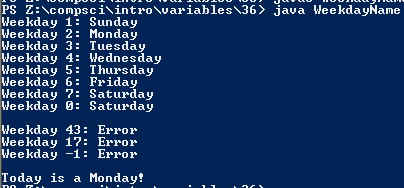# Assignment #36

## Code

```    /// Gian Adoremos
/// Period: 7
/// Program Name: ThirtySixth Program
/// File Name: WeekdayName.java
/// Date Finished: 11/9/2015

import java.util.GregorianCalendar;

public class WeekdayName
{
public static String weekday_name( int weekday )
{
String result = "";

if ( weekday == 1 )
{
result = "Sunday";
}
else if ( weekday == 2 )
{
result = "Monday";
}
else if ( weekday == 3 )
{
result = "Tuesday";
}
else if ( weekday == 4 )
{
result = "Wednesday";
}
else if ( weekday == 5 )
{
result = "Thursday";
}
else if ( weekday == 6 )
{
result = "Friday";
}
else if ( weekday == 7 )
{
result = "Saturday";
}
else if ( weekday == 0 )
{
result = "Saturday";
}
else if ( weekday == 43 )
{
result = "Error";
}
else if ( weekday == 17 )
{
result = "Error";
}
else if ( weekday == -1 )
{
result = "Error";
}
return result;
}

public static void main( String[] args )
{
System.out.println( "Weekday 1: " + weekday_name(1) );
System.out.println( "Weekday 2: " + weekday_name(2) );
System.out.println( "Weekday 3: " + weekday_name(3) );
System.out.println( "Weekday 4: " + weekday_name(4) );
System.out.println( "Weekday 5: " + weekday_name(5) );
System.out.println( "Weekday 6: " + weekday_name(6) );
System.out.println( "Weekday 7: " + weekday_name(7) );
System.out.println( "Weekday 0: " + weekday_name(0) );
System.out.println();
System.out.println( "Weekday 43: " + weekday_name(43) );
System.out.println( "Weekday 17: " + weekday_name(17) );
System.out.println( "Weekday -1: " + weekday_name(-1) );

GregorianCalendar cal = new GregorianCalendar();
int dow = cal.get(GregorianCalendar.DAY_OF_WEEK);

System.out.println( "\nToday is a " + weekday_name(dow) + "!" );
}

}
```

### Picture of the output